Courses

# Design of Joints with Variable Loading Mechanical Engineering Notes | EduRev

## Mechanical Engineering : Design of Joints with Variable Loading Mechanical Engineering Notes | EduRev

The document Design of Joints with Variable Loading Mechanical Engineering Notes | EduRev is a part of the Mechanical Engineering Course Machine Design.
All you need of Mechanical Engineering at this link: Mechanical Engineering

Variable loading in mechanical joints:
Machine parts are often subjected to variable loading. In many cases pulsating or intermittent loads are applied from outside, for example, in punching press forces of very large magnitude is applied for a short while (impulsive force), in crank shafts variable loads act due to nature of force arising from combustion cycle in cylinders. Often dynamic forces appear in the moving parts, e.g., inertia forces in machines and mechanisms, forces due to unbalance of the rotating components etc. Since these forces are to be withstood by the joints, care should be taken while designing a joint capable of resisting adequate load of variable magnitude. Design of two important mechanical joints is discussed below, namely, bolted and welded joints.

2. Bolted joints with variable loading:
Consider design of bolts to fasten a flat cover to a cylinder as shown in figure 11.3.1. In order to ensure leak proofness necessary pretension (usually 2840 d , in Newton while the nominal bolt diameter d is measured in millimeter) is applied. Depending upon operating condition the pressure inside the closed cylinder is likely to vary in somewhat periodic manner. Let the minimum and maximum value of the pressure be pmin and pmax , respectively.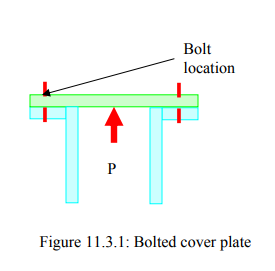The pressure causes external force of magnitude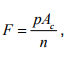n = , where
n= number of equally spaced bolts on the bolt circle
Ac = area of cross section of the cylinder
p = fluid pressure inside the cylinder.

It is known that only a fraction of external load is responsible for tensile stress within bolts, that is

Fb =  F1 + CF

where
F1 = initial tension in the bolt
C = factor that depends on the nature of joints. Some representative values of C’s are tabulated in Table 1 below.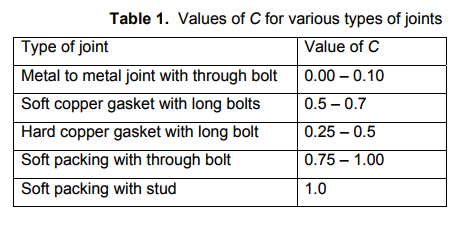Due to fluctuating external force the tensile load within each bolt takes minimum and maximum value of

Fb ,min = Fi + CFmin = Fi + CFmax    and  Fb ,max  = Fi + CFmax

respectively. The average and the fluctuating component of the normal stress are given by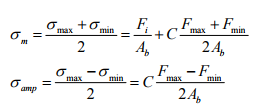respectively, where Ab is the root area of each bolt. The advantage of initial pretension is at once visible from the above expressions. The ratio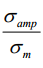gets drastically reduced, The safe size of the bolt can be calculated now from wellknown Soderberg equation given below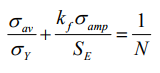where σY = Yield stress of the bolt material,
SE= Corrected endurance limit taking load-, size-, surface finishfactors

N = Factor of safety
k = fatigue stress concentration factor.

Alternatively, Goodman’s equation or Gerber’s line may be used to calculate the root area and hence the size of the bolts. The fatigue stress concentration factor plays an important role in the design. These are found by doing extensive experimentation. A few figures are shown in Table 2.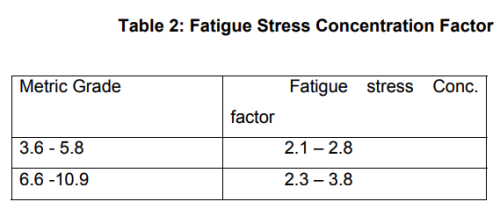3. Welded joints with variable loading: Because of many intricacies involved in design of a welded joint, codes are extensively used to design such joint when it experiences variable loading. The value of the maximum fluctuating load is not allowed to exceed a limit specified in the code. This value depends on
a. type of the joint
b. type of stress experienced by the joint
c. a load factor K defined as the ratio of the minimum stress to the maximum stress. When the load is a steady one the factor takes unit value. For a complete reversal of stress the value of K = -1.

The design stress for completely reversing load is calculated using the formula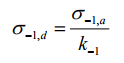where σ −1,d = design stress for complete reversal of stress
σ −1,= allowable fatigue stress
k-1  = fatigue stress concentration factor tabulated below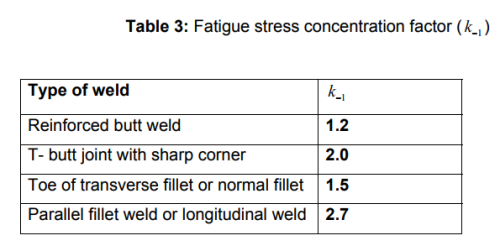The values of the allowable fatigue stress (σ −1,a ) are also tabulated in the design code for various weld geometries. For example, the allowable fatigue stress for fillet weld is given (assuming the weld to be a line) as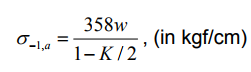where w denotes the leg size of the fillet weld measured in centimeter. The design is found to be safe if the maximum value of the fluctuating stress is found to be lesser than the design stress.

Offer running on EduRev: Apply code STAYHOME200 to get INR 200 off on our premium plan EduRev Infinity!

## Machine Design

57 videos|71 docs|102 tests

,

,

,

,

,

,

,

,

,

,

,

,

,

,

,

,

,

,

,

,

,

;